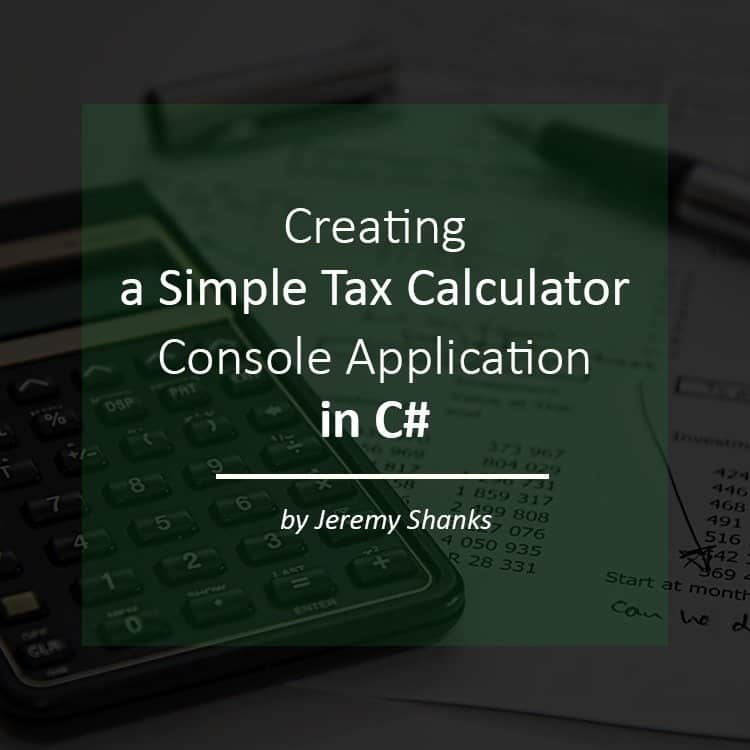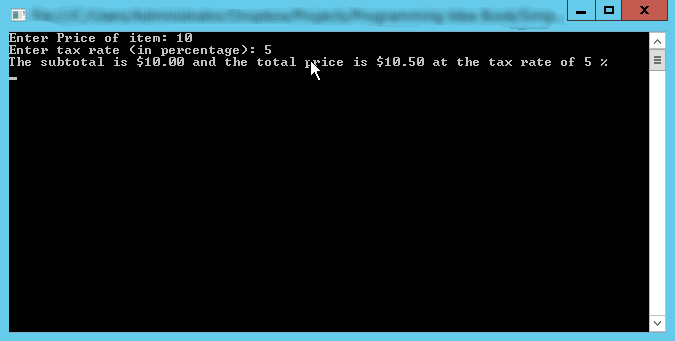# Creating a Simple Tax Calculator Console Application in C#In this tutorial, I will show you how to create a simple tax calculator in C# using Visual Studio. I will show you how to get input from a user and do some calculations on that input. By the end of this tutorial, you will be able to run your tax calculator to calculate the grand total of an item given a specific tax rate.

## Requirements

Difficulty: Easy

Concepts Used:

We want to create an application that can calculate the sales tax for an item based on input from the user. We will then output the subtotal and grand total based on the item amount and tax amount.

First, we are going to start off with a simple console application that calculates the grand total of one item and its tax amount. Then in the next tutorial, we are going to step it up a notch and build a full GUI (Graphical User Interface) for our app and calculate the tax based on more than one item. We will add the ability to calculate different items at different tax rates. For example, food items will be taxed at a different rate than general merchandise.

## Let’s Get Started

To get started, we need to create an empty C# console application in Visual Studio. For more information on how to do this, check out my walkthrough for creating a new console application in Visual Studio.

Now that we have our new blank console application we are ready to get started. The first thing we need to do is get the price of the item and tax rate. We will do that with the following code in the Main method of our application which is the starting point of all C# console applications.

In the code above we are using Console.Write();  instead of Console.WriteLine();  because we do not want to put the cursor on the next line when the user gives input. One thing you will notice is that Console.ReadLine();  gives us a string with will have to be converted to a number later.

Now that we are getting input from the user we are going to do the calculation so that we can give the subtotal and grand total back to the user. To make sure we are following good coding practice we are going to create a separate TaxCalculator class specifically for calculating and outputting the subtotal and grand total when given the input from the above code. Take a look at the following code to see how we go about doing this.

Now let’s go through the above code line by line. First off we create three variables of type decimal to hold the item price, tax rate, and total price. These are created as private variables because we do not want these to be able to be set outside of the TaxCalculator class, they should only be set from inside the class. Note how the names of these variables start with an underscore. This is a common convention to indicate they are private variables for that class. This does nothing for the .NET compiler and is is purely for easier reading.

Next, we have the TaxCalculator constructor which is a method that gets run when the TaxCalculator object is created. This constructor takes in two parameters of type string because this is what we get from the user using Console.ReadLine(); . Inside the constructor, we are parsing the two strings to convert them to type decimal and then assigning them to the private variables in the TaxCalculator class.

You will notice we are dividing the tax rate input from the user by 100. This is to convert the number to a percentage expressed as a decimal. For example, 5% expressed as a decimal is .05

Now we have the CalculateTotalPrice method that does exactly what you would expect, it calculates the total price of the item and assigns it to the private static variable in the TaxCalculator class. C# follows the order of operations here and does the calculation in the parenthesis first and then the addition.

Lastly, we have the GetTotalMsg method that just outputs the subtotal, grand total, and tax rate as a sentence using Console.WriteLine(); . In the string, we are formatting the item price and total price as currency by using :C  and the tax rate as a percentage to two decimal places by using :P2

Now back up to the Main method. Now that we have a fully functional TaxCalculator class from which we can create objects, we need to create a new TaxCalculator object and call the methods in that object. Take a look at the following code to be placed in the Main method.

In the three lines of code above we are creating a new TaxCalculator object called “myTaxCalculator” and passing in the item price and tax rate to the constructor. We are then calling the CalculateTotalPrice method which will calculate the grand total of the item. Last, we are calling the GetTotalMsg method which will output a message with the subtotal, grand total, and tax rate as a percentage.

Side Note, when we run this application later in this tutorial we want the application to pause before closing so that we can see the output. To achieve this we will insert Console.ReadKey();  to the end of the Main method. This will force the application to stop and wait until the user presses a key on the keyboard before closing.

## See it in Action

Now that we have the full application coded, it is time to run it. To run this application in Visual Studio, we click “Start Without Debugging” in the Debug menu as shown below or by pressing Ctrl + F5  to run without debugging.When this application runs it will like shown below.Congratulations! You now have a working tax calculator to calculate the tax for an item. But even better you know how to get input from a user and do some calculations on that input all while utilizing objects and their methods in C#.

Remember I am documenting my journey of learning C# by writing these tutorials, so if you see a mistake or know a better way of doing it then, please let me know in the comments below. Also, if you have any questions be sure to ask in the comments section below and I will do my best to answer.

Be sure to check out my next tutorial when it comes out to see how to create a more advanced version of our tax calculator that can handle more than one item and has a GUI. I will place a link to it here when it is posted. Edit: You can check out my tax calculator with a user interface here.

### About the Author Jeremy Shanks

Hi, my name is Jeremy Shanks. I am a Software Engineer in Raleigh, NC. Recently I embarked on the journey of teaching others how to develop projects in C#. Be sure to check them out if you are new to C#.

follow me on: